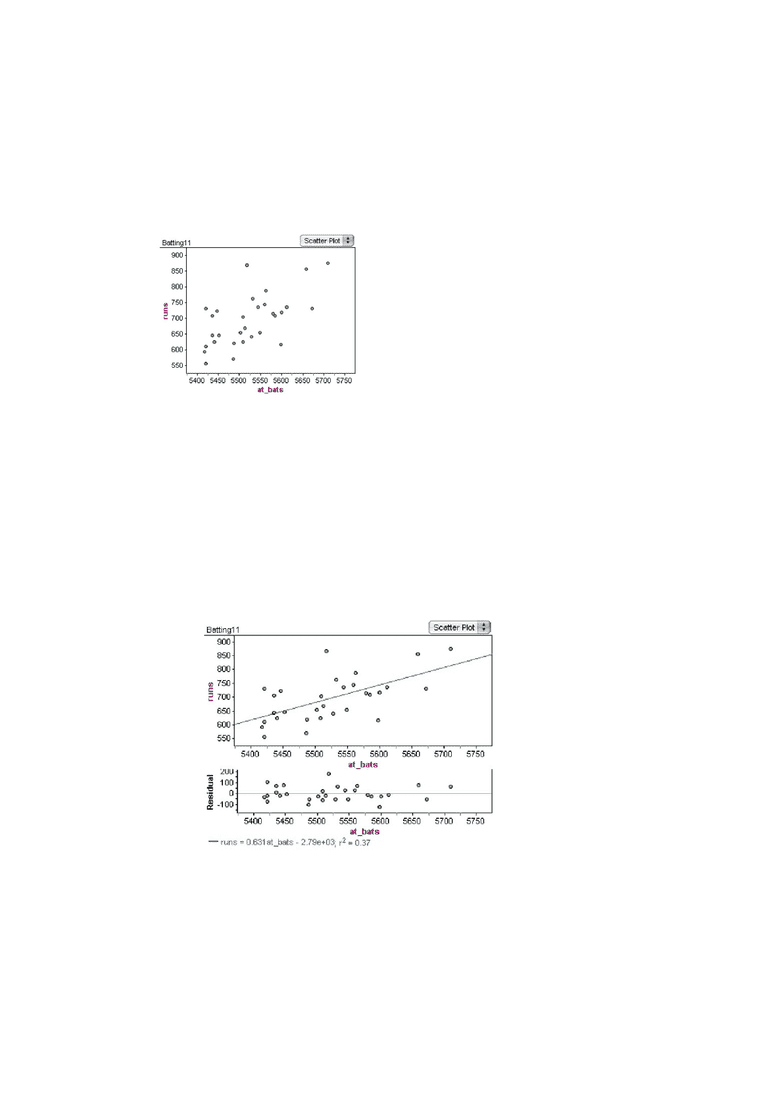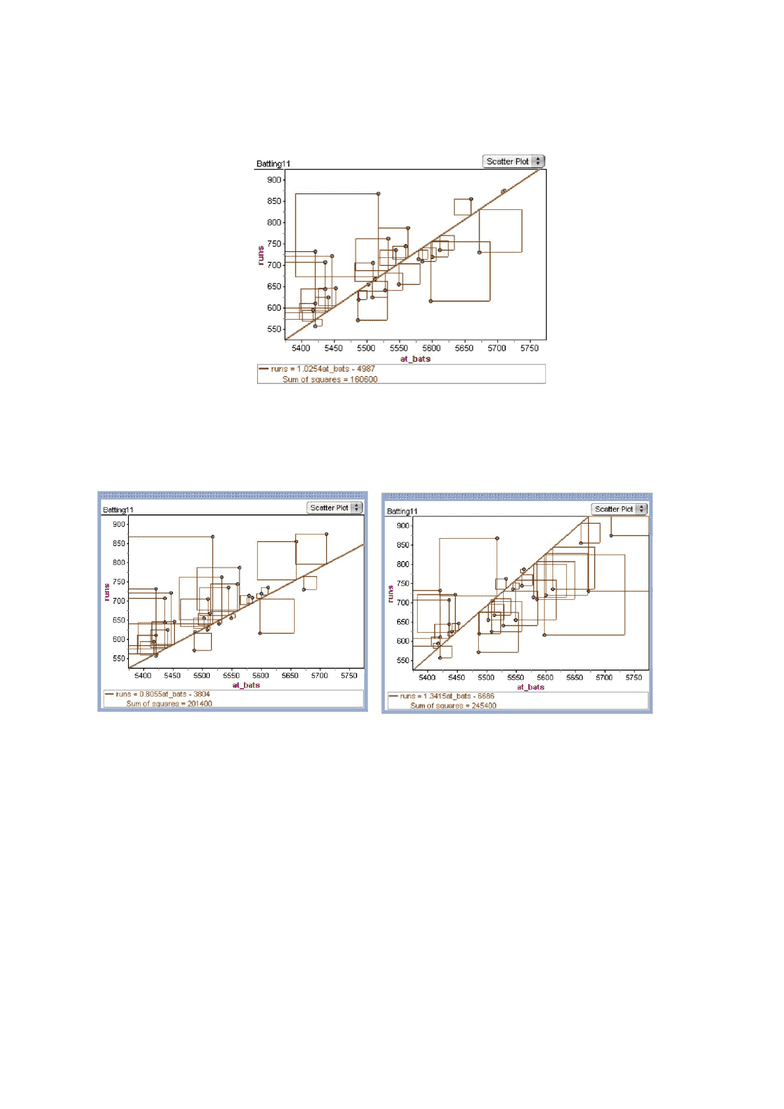Textbook Notes (280,000)
US (110,000)
UCLA (2,000)
STATS (30)
Cha, M (1)
Chapter 4

# STATS 10 Chapter Notes - Chapter 4: Batter Up, Scatter Plot, Dependent And Independent Variables

Department
Statistics
Course Code
STATS 10
Professor
Cha, M
Chapter
4

This preview shows pages 1-2. to view the full 7 pages of the document.Pratishta Natarajan
UID: 504786348
Section: 1A
Statistics Lab 2- Batter Up
1) I have attached the graph for at_bats and runs below:
      From the graph we can see that as the number of at_bats increases, the number of runs
increases as well. So this graph shows us that the relation is positive. Even though the graph
increases overall, it does not increase uniformly, ie: the variables are only moderately
correlated. So from this graph we can see that this variable not the most accurate to predict
the number of runs because we can only conclude that if the number of at_bats are higher,
the team’s runs are higher too. We cannot predict the exact number of runs a team would
score by only looking at the number of at_bats.
2)  I have attached the residual plot below:
The relationship between at_bats and run is linear. The plots are randomly scattered across
the horizontal axis. Therefore a linear model is more appropriate to represent the data.
In addition, the points do not form a “U” or and “Inverted U” shape. Therefore we can see
that a non-linear model would not be appropriate to explain the data.

Only pages 1-2 are available for preview. Some parts have been intentionally blurred.3) I have attached the graph for where I would put a single movable line, if I had to
summarize the graph. I have also added the “Sum of Squares” to it
The sum of squares is- 160600
Now I have attached two other graphs, that do not fit the data as well as the first graph does:
We can see that the sum of squares for these graphs are 201400 and 245400 respectively.
So we can see that the sum of squares reduces, and is the least when the line best fits the
data.
This is because the sum of squares is the square of the spread between each data point
from the mean. Since we are trying to find a line that fits the data the best, the spread needs
to be as little as possible. So the line of best fit will minimize the sum of squares. Which is
why the first graph has the least possible sum of squares for this data.
4) Below I have attached, the duplicate of the graphs I have used in the previous question. I
have changed the “Movable Line” to the “Least Squares Line”